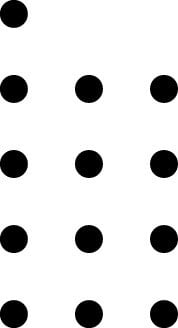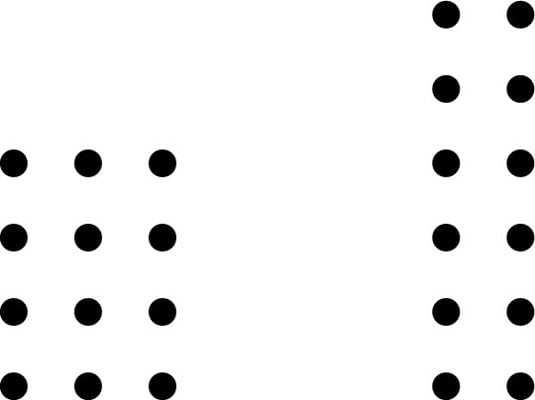##### Basic Math & Pre-Algebra All-in-One For Dummies (+ Chapter Quizzes Online)A prime number can be divided by only itself and 1. This makes it stubborn. Like certain people you may know, a prime number resists being placed in any sort of a box. Look at how the number 13 is depicted in the following figure, for example.Try as you may, you just can’t make a rectangle out of 13 objects. (That may be one reason the number 13 got a bad reputation as unlucky.) The composite numbers can all be placed in rectangular patterns.The number 12 is a composite number because you can place 12 objects in rectangles of two different shapes, as shown above.

Here are all the prime numbers less than 20:

2 3 5 7 11 13 17 19

Now here are all the composite numbers less than 20:

4 6 8 9 10 12 14 15 16 18

As you can see, the list of prime numbers fills the gaps left by the composite numbers. Therefore, every counting number is either prime or composite. The only exception is the number 1, which is neither prime nor composite.